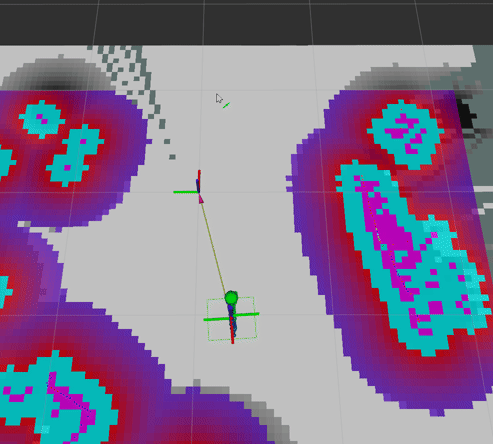### Repository Summary

 Checkout URI https://github.com/FastSense/mppic.git VCS Type git VCS Version master Last Updated 2021-10-13 Dev Status DEVELOPED CI status No Continuous Integration Released RELEASED Tags No category tags. Contributing Help Wanted (0) Good First Issues (0) Pull Requests to Review (0)

Name Version
mppic 0.2.1

# MPPI Local Planner## Overview

Navigation2 Controller plugin. Currently tested on ros2 foxy.

This is a controller (local trajectory planner) that implements model predictive path integral control to track a path with collision avoidance.

The main idea of the algorithm is to sample batch of consecutive controls (linear and angular velocities) by given time window and dt, use model to predict real velocities (linear and angular) for each time_step by given controls and initial velocities (current Twist), this can be explained as follows V( t+1 ) = F( X(t) ), where - V(t+1) - velocities (linear and angular) of all batches at time step t + 1, shape [batch size x 2] - X(T) - batches of time step t, consisting current velocities (linear, angular), controls (linear, angular) and dt, shape [batch size x 5]

then integrate these velocities to get trajectories, evalueate cost for each trajectory, and take relative scale of original controls (mean of batches controls with overweight for those controls which propagated trajectories has lesser cost) using softmax function calculated with costs.

This implementation has reworked cost function, which for now has 4 components which are related to obstacle avoidance, goal following, reference alignment, goal angle considerring near the goal. May be i'll "pluginize" this in future like in dwb There is no GPU acceleration / parallelisation, but you can implement generic "model" function which uses neural network (which predict linear and angular speed of the robot by given control and current state (curent linear and angular velocities)) which can opperate on whole batch in parrallel. (i'll probably implement this in near future)

This plugin implements the nav2_core::Controller interface allowing it to be used across the navigation stack as a local trajectory planner in the controller server's action server (controller_server).

## Dependencies

MPPIc package requires a modern C++ compiler supporting C++17, and Conan C++ package manager:

``````pip install conan

``````

## Configuration

Parameter Type Definition
iteration_count int Iteration count in MPPI algorithm
lookahead_dist double Max lenght of the global plan, considering by local planner
batch_size int Count of randomly sampled trajectories
time_steps int Number of points propagated in time in each sampled trajectory
model_dt double Time interval between two points in sampled trajectories
v_std double Standart deviation for linear speed sampling
w_std double Standart deviation for angular speed sampling
v_limit double Linear speed control limit
w_limit double Angular speed control limit
temperature double Selectiveness of trajectories by their costs (The closer this value to 0, the more we take controls with less cost), 0 mean use control with best cost, huge value will lead to just taking mean of all trajectories withou cost consideration
goal_weight double
goal_power int
reference_cost_weight double
reference_cost_power int
obstacle_cost_weight double
obstacle_cost_power int
goal_angle_cost_weight double
goal_angle_cost_power int
inflation_cost_scaling_factor int Must be set accurately according to inflation layer params
inflation_radius double Must be set accurately according to inflation layer params
threshold_to_consider_goal_angle double Minimal distance between robot and goal above which angle goal cost considered
approx_reference_cost bool Use approximate point to segment distance calculation
visualize bool Use visualization

Example fully-described XML with default parameter values:

``````controller_server:
ros__parameters:
use_sim_time: True
controller_frequency: 10.0
min_x_velocity_threshold: 0.001
min_y_velocity_threshold: 1.0
min_theta_velocity_threshold: 0.001
progress_checker_plugin: "progress_checker"
goal_checker_plugin: "goal_checker"
controller_plugins: ["FollowPath"]

progress_checker:
plugin: "nav2_controller::SimpleProgressChecker"
movement_time_allowance: 10.0

goal_checker:
plugin: "nav2_controller::SimpleGoalChecker"
xy_goal_tolerance: 0.25
yaw_goal_tolerance: 0.25
stateful: True

FollowPath:
plugin: "mppi::Controller<float>"
time_steps: 20
model_dt: 0.1
batch_size: 300
v_std: 0.1
w_std: 0.3
v_limit: 0.5
w_limit: 1.3
iteration_count: 2
temperature: 0.25
reference_cost_power: 1
reference_cost_weight: 5
goal_cost_power: 1.0
goal_cost_weight: 20.0
goal_angle_cost_power: 1.0
goal_angle_cost_weight: 5.0
obstacle_cost_power: 2
obstacle_cost_weight: 10
inflation_cost_scaling_factor: 3
threshold_to_consider_goal_angle: 0.3
approx_reference_cost: false

``````

## Topics

Topic Type Description
`trajectories` `visualization_msgs/MarkerArray` Randomly generated trajectories, including resulting control sequence
`transformed_global_plan` `nav_msgs/Path` Part of global plan considered by local planner

AutoRally

### CONTRIBUTING

No CONTRIBUTING.md found.